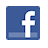Games
Problems
Go Pro!

# Directionally Challenged

Pro Problems > Math > Number and Quantity > Number Theory > Patterns

## Directionally Challenged

Given the following sequence of compass directions, state the next two directions in the sequence and explain your answer.

North, East, West, South, South, …

Presentation mode
Problem by allie

## Solution

In order to make it feasible for teachers to use these problems in their classwork, no solutions are publicly visible, so students cannot simply look up the answers. If you would like to view the solutions to these problems, you must have a Virtual Classroom subscription.Assign this problem

## Similar Problems

### Letter Sequence

Given the following sequence, what are the next two letters in the sequence?

D, H, L, P, S,...

### Predict Y

Predict the value of y in the following chart:

x
y
1
0
2
3
3
8
4
15
10
?

### Find the Pattern

Given the following table of x and y values, find a pattern relating the two numbers, and then answer the question below.

X
Y
231
6
513
15
228
32
732
42
449
144

If all x values are three digit numbers, what is the difference between the largest possible y and the smallest possible y?

### Triangular Numbers

A triangular number is a number which can be represented by a set of dots drawn in a triangular pattern. For example, ten is a triangular number because it can be displayed like this:

```♦ ♦ ♦ ♦
♦ ♦ ♦
♦ ♦
♦
```

Thus, the first few triangular numbers are 1, 3, 6, 10, and 15. Find the 200th triangular number.# Featured Resources on This Site

Create Christmas ornaments and more
Print fun mazes in many different shapes

# Blogs on This SiteReviews and book lists - books we love!The site administrator fields questions from visitors.Like us on Facebook to get updates about new resources